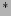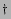Next: Introduction

# Probability densities and preservation of randomness in wave turbulence Physics Letters A, 332, 230, (2004).

Yeontaek Choi, Yuri V. Lvovand Sergey NazarenkoMathematics Institute, The University of Warwick, Coventry, CV4 7AL, UKDepartment of Mathematical Sciences, Rensselaer Polytechnic Institute, Troy, NY 12180

### Abstract:

Turbulence closure for the weakly nonlinear stochastic waves requires, besides weak nonlinearity, randomness in both the phases and the amplitudes of the Fourier modes. This randomness, once present initially, must remain over the nonlinear evolution time. Finding out to what extent is this true is the main goal of the present Letter. For this analysis we derive an evolution equation for the full probability density function (PDF) of the wave field. We will show that, for any statistics of the amplitudes, phases tend to stay random if they were random initially. If in addition the initial amplitudes are independent variables they will remain independent in a coarse-grained sense, i.e. when considered in small subsets which are much less than the total set of modes.Next: Introduction
Dr Yuri V Lvov 2007-01-17